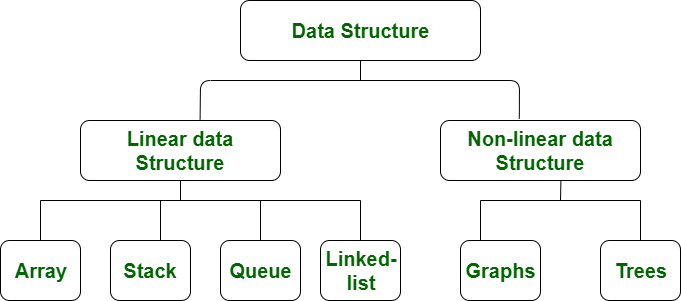# Difference between Linear and Non-linear Data Structures

Linear Data Structure:
Data structure where data elements are arranged sequentially or linearly where the elements are attached to its previous and next adjacent in what is called a linear data structure. In linear data structure, single level is involved. Therefore, we can traverse all the elements in single run only. Linear data structures are easy to implement because computer memory is arranged in a linear way. Its examples are array, stack, queue, linked list, etc.

Non-linear Data Structure:
Data structures where data elements are not arranged sequentially or linearly are called non-linear data structures. In a non-linear data structure, single level is not involved. Therefore, we can’t traverse all the elements in single run only. Non-linear data structures are not easy to implement in comparison to linear data structure. It utilizes computer memory efficiently in comparison to a linear data structure. Its examples are trees and graphs.Difference between Linear and Non-linear Data Structures:

S.NO Linear Data Structure Non-linear Data Structure
1. In a linear data structure, data elements are arranged in a linear order where each and every elements are attached to its previous and next adjacent. In a non-linear data structure, data elements are attached in hierarchically manner.
2. In linear data structure, single level is involved. Whereas in non-linear data structure, multiple levels are involved.
3. Its implementation is easy in comparison to non-linear data structure. While its implementation is complex in comparison to linear data structure.
4. In linear data structure, data elements can be traversed in a single run only. While in non-linear data structure, data elements can’t be traversed in a single run only.
5. In a linear data structure, memory is not utilized in an efficient way. While in a non-linear data structure, memory is utilized in an efficient way.
6. Its examples are: array, stack, queue, linked list, etc. While its examples are: trees and graphs.

My Personal Notes arrow_drop_upCheck out this Author's contributed articles.

If you like GeeksforGeeks and would like to contribute, you can also write an article using contribute.geeksforgeeks.org or mail your article to contribute@geeksforgeeks.org. See your article appearing on the GeeksforGeeks main page and help other Geeks.

Please Improve this article if you find anything incorrect by clicking on the "Improve Article" button below.